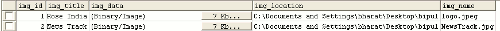Tutorials

# How To Retrieve Image From From MySQL Using Java

In this section we will discuss about how to retrieve image from the MySQL using Java.

In this section we will discuss about how to retrieve image from the MySQL using Java.

## How To Retrieve Image From From MySQL Using Java

In this section we will discuss about how to retrieve image from the MySQL using Java.

This example explains you about all the steps that how to retrieve image from MySQL database in Java. To retrieve an image from the database we will have to run the "select" SQL query.

To retrieve an image from the database we can use the following line of code where fetching the resultset as follows :

```Blob img = rs.getBlob(columnIndex);
InputStream is = img.getBinaryStream()```

Or you can directly use the following line of code where fetching the resultset as follows :

```InputStream is = rs.getBinaryStream(columnIndex);
```

Example

Here I am giving a simple example which will demonstrate you about how to retrieve image from the database. In this example we will first create a database table where we will insert the image into the table (How Read Here). Then we will create a Java class where we will write the code for connecting the database and then we will write a SQL query for searching the records into the database table and then we will get the image value using rs.getBinaryStream(columnIndex). Further for the convenience to get the image detail we will read the image using read() method of ImageIO class and kept this image into the BufferedImage and next we will find the image width and height.

Source Code

RetrieveImageFromDB.java

```import java.awt.image.BufferedImage;
import java.io.InputStream;
import java.sql.Connection;
import java.sql.DriverManager;
import java.sql.PreparedStatement;
import java.sql.ResultSet;

import javax.imageio.ImageIO;

public class RetrieveImageFromDB {

public static void main(String args[])
{
String className = "com.mysql.jdbc.Driver";
String url = "jdbc:mysql://localhost/record";
String user = "root";
Connection con = null;
PreparedStatement ps = null;
InputStream is = null;
try
{
Class.forName(className);
System.out.println("img_id \t img_title \t \t img_location \t \t \t \t \t img_name \t WidthXHeight");
ps = con.prepareStatement("select * from image");
ResultSet rs = ps.executeQuery();
while(rs.next())
{
int img_id = rs.getInt(1);
String img_title = rs.getString(2);
String img_location = rs.getString(4);
String img_name = rs.getString(5);
is = rs.getBinaryStream(3);
int width = bimg.getWidth();
int height = bimg.getHeight();
System.out.println(img_id + " \t " + img_title+ " \t " + img_location+ " \t " + img_name+ " \t "+ width+"X"+height);
}
rs.close();
is.close();
}
catch(Exception e)
{
e.printStackTrace();
}
}
}
```

Output

When you will execute the above code and let there are two records in the database table then you will get the output as follows :

The database table is as follows :And the output will be as follows :